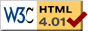### Journée-séminaire de combinatoire

#### (équipe CALIN du LIPN, université Paris-Nord, Villetaneuse)

Le 04 juin 2019 à 14h00 en B107, Antonio Jimenez Pastor nous parlera de : A Computable Extension for Holonomic Functions: DD-Finite Functions

Résumé : D-finite functions is a well known class of functions. They are defined via a linear differential equation with polynomial coefficients and we can find many examples in elementary functions (exp(x), sin(x)), combinatorial generating functions (all P-recursive sequence have a D-finite generating function) and many other special functions. In this talk we will see a natural extension to this class: the DD-finite (and, more generically, Dn-finite) functions. These functions are defined using a linear differential equation with D-finite coefficients. We will described the structural properties known for this larger class of functions.

 Dernière modification : Monday 24 January 2022Contact pour cette page : Cyril.Banderier at lipn.univ-paris13.fr